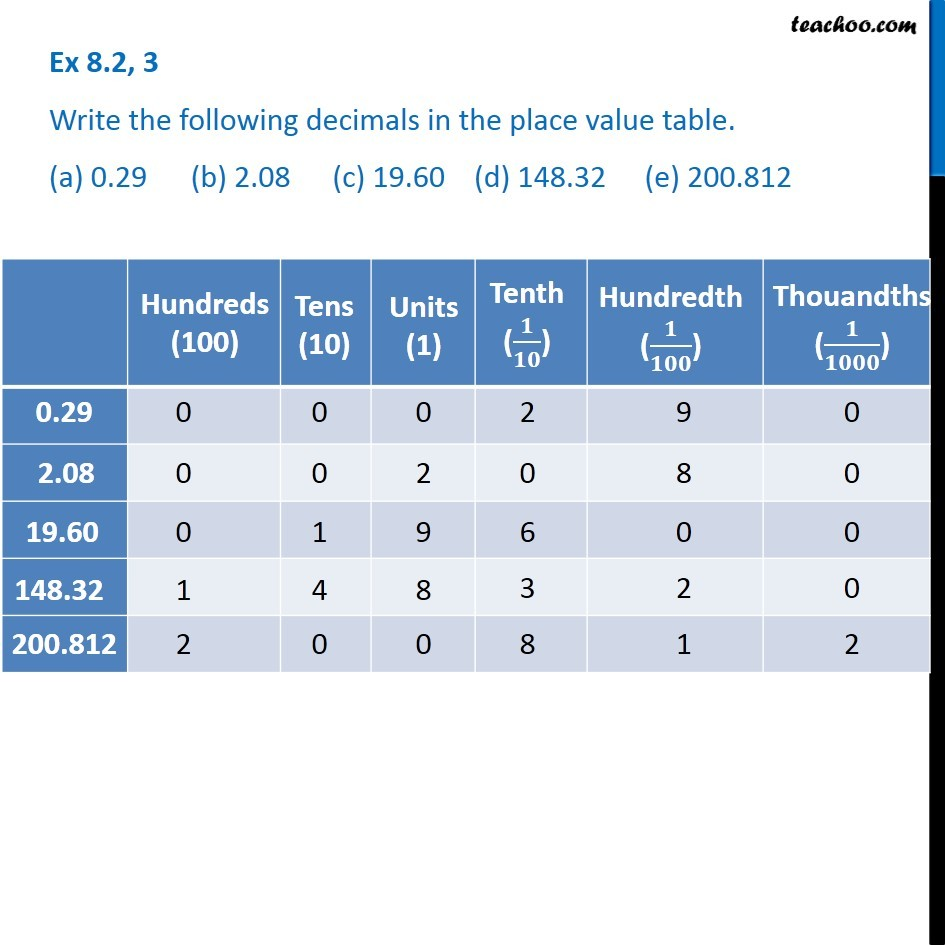Writing Fractions as Decimals worksheet

Chapter 8 Class 6 Decimals
Serial order wiseLearn in your speed, with individual attention - Teachoo Maths 1-on-1 Class

### Transcript

Question 3 - Chapter 8 Class 6 NCERT Books Write the following decimals in the place value table. (a) 0.29 (b) 2.08 (c) 19.60 (d) 148.32 (e) 200.812 For (a) 0.29 Hundreds (100) = 0 Tens (10) = 0 Units (1) = 0 Tenths (1/10) = 2 Hundredth (1/100) = 9 Thousandths (1/1000) = 0 For (b) 2.08 Hundreds (100) = 0 Tens (10) = 0 Units (1) = 2 Tenths (1/10) = 0 Hundredth (1/100) = 8 Thousandths (1/1000) = 0 For (c) 19.60 Hundreds (100) = 0 Tens (10) = 1 Units (1) = 9 Tenths (1/10) = 6 Hundredth (1/100) = 0 Thousandths (1/1000) = 0 For (d) 148.32 Hundreds (100) = 1 Tens (10) = 4 Units (1) = 8 Tenths (1/10) = 3 Hundredth (1/100) = 2 Thousandths (1/1000) = 0 For (e) 200.812 Hundreds (100) = 2 Tens (10) = 0 Units (1) = 0 Tenths (1/10) = 8 Hundredth (1/100) = 1 Thousandths (1/1000) = 2